# Triangular Numbers

Today I gave a math lesson to my five year old and stumbled upon a mathematical property that I didn’t know of: any square number is a sum of two triangular numbers.

During the lesson, I used these two toys: Subtraction Abacus by Melissa & Doug and MathLink Cubes by Learning Resources. Here they are: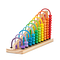Subtraction Abacus by “Melissa & Doug”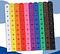MathLink Cubes by “Learning Resources”

What are triangular numbers? That is the arrangement of the beads on the Subtraction abacus: it’s a series in which each term is one larger than the preivous one. They form a triangle, and this is the reason why the Subtraction Abacus has a triangular shape. It is easier to see this when a quantity is made to look like a pyramid: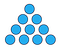Every row is one more than the previous

Using the MathLink Cubes, I presented it like this: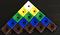15 is a triangular number

The interesting thing to note is that a square number can be split into two such triangular numbers,

Notice that one of the triangles will always be smaller by one row than the larger one.

We can lie the triangular shape on its side in order to make it easier to write on grid paper.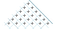Triangular number on grid paper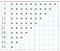The first 10 triangular numbers

Compare this list with the first 10 square numbers: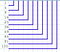The first 10 square numbers

(An interesting observation is that 36 is both a triangular number and a square number because it appears in both lists.)

We can see that the square number 9 equals the sum of triangular numbers 3 and 6. Likewise, the square number 36 equals the sum of triangular numbers 15 and 21. We can match them like this,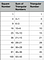Square number as the sum of two triangular numbers

From the table above, as well as the photo above, we can see that the same triangular number appears in two adjacent squares in the list. For example, triangular number 15 appears in both squares 25=15+10 an 36=21+15. In the smaller square it is the larger triangle, and in the bigger square it is the smaller triangle. The difference 36–25 between them equals the sum of triangular numbers 10+21, and the triangular number 15 disappears from the expression.

This gives us an idea that adding and subtracting squares from each other should “cancel out” a lot of terms. Indeed, the following table shows that the alternating sum of the first n squares equals to the n-th triangular number.

Algebraically, we can derive it like this:

If we subtract and add alternatingly these lines, we will get:

In mathematics, a sum of a sequence of terms is called “series.” The n-th triangular number is a series of integers. Combining with the previous result we have shown that a series of integers equals an alternating series of squares. This yields a non-trivial algebraic identity:

There is a familiar formula to calculate a triangular number. It is the formula for the sum of the first n consecutive integers. It is n(n+1)/2, and it is easy to derive it from the Subtraction Abacus.Subtraction Abacus

If we pair the first arch with the last, the second with the second-last, and so on, we will see that each pair adds to 11. Using this observation we quickly calculate that the total number of beads is 11*5, or 55. The pattern is (n+1)*(n/2) where n was 10.

What about when n is odd? Let’s sum up to the ninth arch only. We can form four pairs, each of which sums to a ten: 9+1, 8+2, 7+3, 6+4. We can not pair the fifth arch with anything because we run out of arches. The pattern appears to be (n+1)(n-1)/2+(n+1)/2. After factoring (n+1)/2 because it appears in both terms, we see that the formula simplifies to the same n(n+1)/2.

(The fact that a triangular number can be calculated using the formula n(n+1)/2 means that it can never be a prime number.)

Given that the n-th triangular number is n(n+1)/2, the previous triangular number is (n-1)n/2. Adding the two consecutive triangular numbers results in a square, as we have previously observed.

Another interesting observation is the fact that the number of ways to choose two items out of n is the (n-1)-th triangular number. The following diagram is a self-explanatory visual proof.

Therefore, because a square is the sum of two consecutive triangular numbers, it can be expressed as,

So a square encodes a number of combinations? Interesting…

I’ll leave it here for you to ponder and experiment.

The course of history is determined by the spreading of ideas. I’m spreading the good ones.

## More from Boris Reitman

The course of history is determined by the spreading of ideas. I’m spreading the good ones.